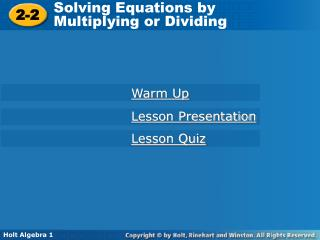DownloadDownload Presentation2-2

# 2-2

Télécharger la présentation## 2-2

- - - - - - - - - - - - - - - - - - - - - - - - - - - E N D - - - - - - - - - - - - - - - - - - - - - - - - - - -
##### Presentation Transcript

1. Solving Equations by Multiplying or Dividing 2-2 Warm Up Lesson Presentation Lesson Quiz Holt Algebra 1

2. 2 3 Warm Up Evaluate each expression (#1-4). 1. (–7)(2.8) 2. 0.96 ÷ 6 3. 4. 19.6 5. Write an equation to represent the relationship. Then solve the equation: 0.16 Ten less than a number is equal to 12. n – 10 = 12 n=22 6. The angles in the diagram are Complementary (add to 90º). Write and solve an equation to find the value of x. 1 x + 42 = 90 x=48

3. Objective Solve one-step equations in one variable by using multiplication or division.

4. Solving an equation that contains multiplication or division is similar to solving an equation that contains addition or subtraction. Use inverse operations to undo the operations on the variable. Multiplication Division Division Multiplication

5. j –8 = 3 –24 3 j –8 = Check 3 –8 Example 1A: Solving Equations by Using Multiplication Solve the equation. Since j is divided by 3, multiply both sides by 3 to undo the division. –24 = j To check your solution, substitute –24 for j in the original equation. –8 –8 

6. n = 2.8 6 n = 2.8 Check 6 16.8 2.8 6 Example 1B: Solving Equations by Using Multiplication Solve the equation. Since n is divided by 6, multiply both sides by 6 to undo the division. n = 16.8 To check your solution, substitute 16.8 for n in the original equation. 2.8 2.8 

7. Check 9y = 108 Example 2A: Solving Equations by Using Division Solve the equation. Check your answer. 9y = 108 Since y is multiplied by 9, divide both sides by 9 to undo the multiplication. y = 12 To check your solution, substitute 12 for y in the original equation. 9(12) 108 108 108 

8. Check –4.8 = –6v Example 2B: Solving Equations by Using Division Solve the equation. Check your answer. –4.8 = –6v Since v is multiplied by –6, divide both sides by –6 to undo the multiplication. 0.8 = v –4.8 –6(0.8) To check your solution, substitute 0.8 for v in the original equation. –4.8 –4.8 

9. Remember that dividing is the same as multiplying by the reciprocal. When solving equations, you will sometimes find it easier to multiply by a reciprocal instead of dividing. This is often true when an equation contains fractions.

10. 6 5 6 5 6 5 6 5 The reciprocal of is . Since w is multiplied by , multiply both sides by . 5 w = 20 Check 6 20 Example 3A: Solving Equations That Contain Fractions Solve the equation. 5 w= 20 6 w = 24 To check your solution, substitute 24 for w in the original equation. 2020 

11. 3 2 1 3 3 8 1 1 3 16 16 8 8 2 The reciprocal of is 8. Since z is multiplied by , multiply both sides by 8. = z 3 16 1 Check = z To check your solution, substitute for z in the original equation. 8 Example 3B: Solving Equations That Contain Fractions Solve the equation. 3 = z 16 

12. 1 Ciro puts of the money he earns from mowing lawns into a college education fund. This year Ciro added \$285 to his college education fund. Write and solve an equation to find how much money Ciro earned mowing lawns this year. 4 Example 4: Application one-fourth times earnings equals college fund Write an equation to represent the relationship. Substitute 285 for c. Since m is divided by 4, multiply both sides by 4 to undo the division. Ciro earned \$1140 mowing lawns. m = \$1140

13. Properties of Equality

14. Properties of Equality

15. Properties of Equality

16. 8 8 = a a 4 4 = c c WORDS Division Property of Equality You can divide both sides of an equation by the same nonzero number, and the statement will still be true. NUMBERS 8 = 8 2 = 2 ALGEBRA a = b (c ≠ 0) Properties of Equality

17. Assignment • L2-2 pg 87 #20-104x4

18. Lesson Quiz: Part 1 Solve each equation. 1. 2. 3. 8y = 4 4. 126 = 9q 5. 6. 21 2.8 –14 40

19. 7. A person's weight on Venus is about his or her weight on Earth. Write and solve an equation to find how much a person weighs on Earth if he or she weighs 108 pounds on Venus. 9 10 Lesson Quiz: Part 2